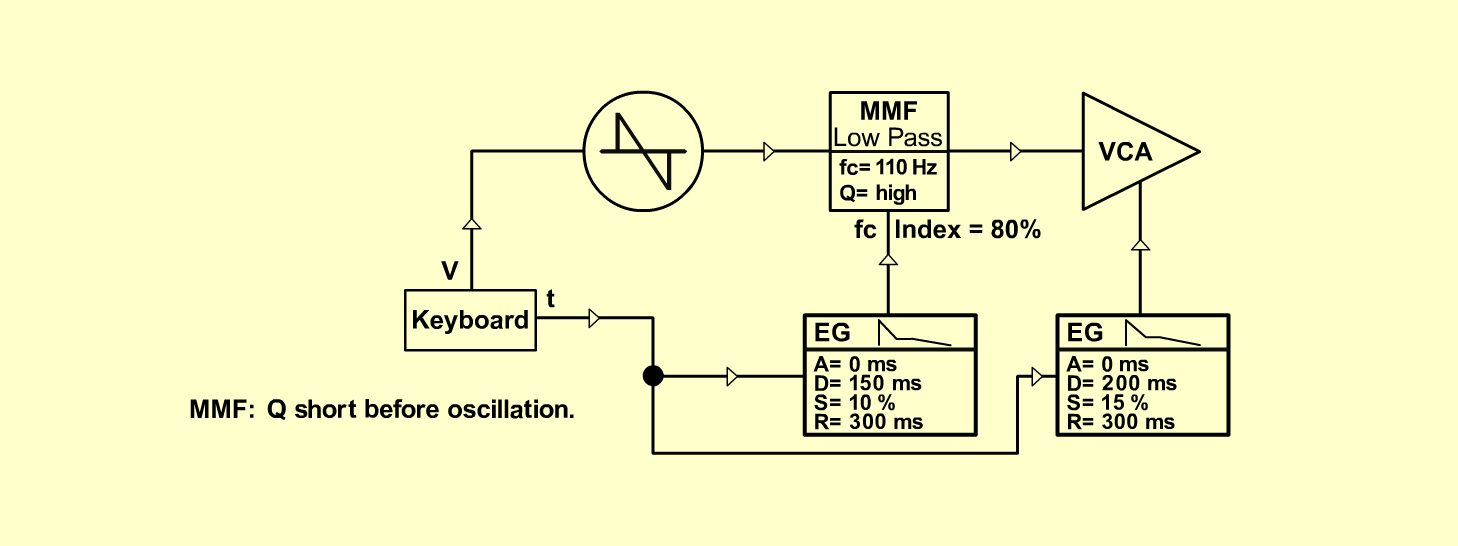EasyResoS y n t hD o e p f e r A - 100 Connections: Settings: EasyReso1: A-111 (Saw)  <=>  A-121 (Audio In)   A-121 (Low)  <=>  A-131 (Audio In 1)   A-140/1 (Output)  <=>  A-121 (FCV 2)   A-140/2 (Output)  <=>  A-131 (CV 1)   A-131 (Audio Out) <=> Amplifier   Trigger/Gate and Pitch-Voltage from keyboard. A-121 (Audio Level = 10, Freq = 4,   FCV 2 = 8, Res = 8)   A-140/1 (A = 0, D = 4, S = 2, R = 5, Range = M)   A-140/2 (A = 0, D = 5, S = 3, R = 5, Range = M)   A-131 (Gain = 0, Audio In 1 = 10, Audio Out = 10) EasyReso2: A-121 (Audio Level = 10, Freq = 4,   FCV 2 = 7.5, Res = 8)   A-140/1 (A = 0, D = 4, S = 1.5, R = 5, Range = M)   A-140/2 (A = 3, D = 5, S = 3, R = 5, Range = M)   A-131 (Gain = 0, Audio In 1 = 10, Audio Out = 10) Notes: Set Q from A-121 short before oscillation.   A-111: The modul A-110 is also possible. Josef MuellerSound samples Pachelbel Canon in D (with dig. effects) Easyreso 1 (65 Hz) Easyreso 1 (261 Hz) Easyreso 2 (65 Hz) Easyreso 2 (261 Hz)